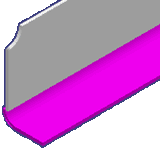# <FlangeGroup> - Intergraph Smart 3D - Administration & Configuration - Hexagon PPM

## Intergraph Smart 3D Tribon Interface

Language
English (United States)
Product
Intergraph Smart 3D
Subproduct
Tribon
Search by Category
Smart 3D Version
13

Defines flange plates at the edge of plates. Flange plates use properties from the parent plate and are created in the same way as the parent plate, as defined for Instance on the panel.<FlangeGroup GroupId="7" BendingRadius ="27.5" Height="114.0" Thickness="8.0">

<Flange CompId="1" Assembly="1063-00_Flange">

<ModelRef ObjType="PlanePanel" ObjId="CG8420-T296F"

CompType="Limit" CompId="3"/>

<Trace>

<StartPoint2d U="1.4450000000E003"

V="8.7520000000E003"/>

<Segment2d>

<Amplitude2d U="0.0000000000E000"

V="0.0000000000E000"/>

<Node2d U="1.4450000000E003"

V="4.9580000000E003"/>

</Segment2d>

</Trace>

<Offset>4.0</Offset>

<Inclination>

<Vector3d U="0.0000000000E000"

V="0.0000000000E000" W="1.0000000000E000"/>

<Vector3d U="0.0000000000E000"

V="0.0000000000E000" W="1.0000000000E000"/>

</Inclination>

</Flange>

.

.

</FlangeGroup>

<Flange>

Contains all definitions for a single flange plate.

<Assembly>

Defines assembly information for the part. This attribute is optional.

<ModelRef>

Identifies an object.

CompId

Defines a unique numeric identifier for the object within the defined value of CompType.

ObjType

Defines an object type, such as PlanePanel, CurvedPanel, or ShellCurve.

ObjId

Defines a unique name for an object. Smart 3D maps ObjId to the Node Type property and uses it to track existing objects during re-import to determine if an object is new.

CompType

Defines the type of feature.

<Trace>

Defines the length and end points of the flange plate.

StartPoint2d

Defines the starting node point coordinates in the 2D U-V plane.

<Segment2d>

Specifies a segment of the contour in the 2D U-V plane. The segment can be a straight line or an arc.

Amplitude2d

Defines the distance of the midpoint of a segment from a line drawn between the node points. The U and V values are 0 for a straight line.

Node2d

Defines the ending node point coordinates of a contour segment in the 2D U-V plane.

<Offset>

Offset of the flange plate from the plate edge.

<Inclination>

Defines the orientation angle of the flange plate.

<Vector3D>

Defines the inclination vectors at a node point on <Trace>. If two values of <Vector3D> are provided for <Inclination>, then the orientation angles are applied at the pillar ends. If more than two values of <Vector3D> are provided, then the orientation angles are applied at each node of the trace.

U

Defines a value along the U-axis of the local coordinate system.

V

Defines a value along the V-axis of the local coordinate system.

W

Defines a value along the W-axis of the local coordinate system.